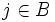Difference between revisions of "Free implies residually finite"

This article gives the statement and possibly, proof, of an implication relation between two group properties. That is, it states that every group satisfying the first group property (i.e., free group) must also satisfy the second group property (i.e., residually finite group)
View all group property implications | View all group property non-implications
Get more facts about free group|Get more facts about residually finite group

Statement

Any free group is a residually finite group, i.e., for every non-identity element of a free group, there is a normal subgroup of finite index in the whole group not containing that element.

Proof

Proof idea

The idea is to use the fact that finite groups are big enough to accommodate a particular word evaluating to a non-identity element.

Proof details

Given: A free group$F$ with freely generating set$T$. A non-identity element$a \in F$.

To prove: There exists a normal subgroup$N$ of$F$ such that$F/N$ is a finite group and$a \notin N$.

Proof: We write:$a = a_na_{n-1} \dots a_2a_1$

as a reduced form expression for$a$ in terms of$T$. Thus, for each$a_i$, either$a_i \in T$ and$a_i^{-1} \in T$. We now define a function$f:T \to S_{n+1}$ where$S_{n+1}$ is the symmetric group on the set$\{1,2,3,\dots,n+1\}$:

•$f(t)$ is the identity element if$t$ is not equal to any of the$a_i$s or their inverses.
• Suppose$A$ is the set of$i$s such that$a_i = t$ and$B$ is the set of$j$s such that$a_j^{-1} = t$. Then, set$f(t)$ as any permutation$\sigma$ that sends each$i \in A$ to$i + 1$, and for each$j \in B$, sends$j + 1$ to$j$. This is well-defined since an element and its inverse cannot occur adjacently in the reduced form expression for a word.

We have thus obtained a function$f:T \to S_{n+1}$. This extends uniquely to a homomorphism from$F$ to$S_{n + 1}$, because$F$ is free. Moreover, under this homomorphism, we see that the image of$a$ sends$1$ to$n + 1$, and is not the identity element. The kernel of this homomorphism is thus a normal subgroup of$F$ with finite quotient group (a subgroup of$S_{n+1}$). Moreover, the kernel does not contain$a$, because the permutation induced by$a$ sends 1 to$n + 1$ (as can be verified by noting that each$a_i$ sends$i$ to$i + 1$, by construction_.